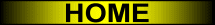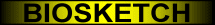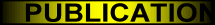A New Way to Represent the Relative Position between Areal Objects
MOVIE
 In PAMI1999, the notion of the histogram of forces is introduced. Such a histogram constitutes a fuzzy qualitative representation of the relative position between two objects. We demonstrate that the force histogram generalizes and supersedes the angle histogram. In particular, the histogram of constant forces coincides with the histogram of angles, but without its weaknesses (long processing times, anisotropy, requirement for raster data...). The two histograms are fundamentally equivalent, even though the first arises from the continuous case and the second from the discrete case. The movie below shows two rectangular objects, and the histograms associated with these objects. Different sets of directions are considered (the number of directions corresponds to the number of values that represent each histogram). The largest set contains 10000 directions and the smallest one only 8. Note that the intrinsic form of the histogram of angles only appears when few directions are considered. The shape of the force histogram is remarkably stable.

MOVIE (zip file, 318KB)